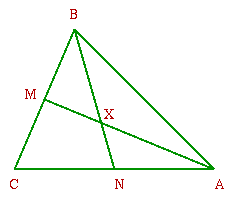#### You may also like### Doodles

Draw a 'doodle' - a closed intersecting curve drawn without taking pencil from paper. What can you prove about the intersections?### Russian Cubes

I want some cubes painted with three blue faces and three red faces. How many different cubes can be painted like that?### Picture Story

Can you see how this picture illustrates the formula for the sum of the first six cube numbers?

# No Right Angle Here

##### Age 14 to 16Challenge Level

This is the solution by Tim from Gravesend Grammar School.

Assume that two of the internal angle bisectors, $AM$ and $BN$, are perpendicular to each other, meeting at $X$, ie $\angle AXB = 90^\circ$.
In triangle $\Delta AXB$, $\angle AXB + \angle BAX + \angle XBA = 180^\circ$
so $\angle BAX + \angle XBA = 90^\circ$.
But $\angle XAC = \angle XAB$ and $\angle ABX = \angle XBC$
so the sum of the angles in the triangle is $2 ( \angle BAX + \angle XBA ) + \angle BCA = 180^\circ + \angle BCA$
so $\angle BCA = 0$, so $ABC$ is not a triangle as it only has two angles,
hence $AM$ and $BN$ are not perpendicular.

Note: What is happening here is that $BC$ is parallel to $AC$.